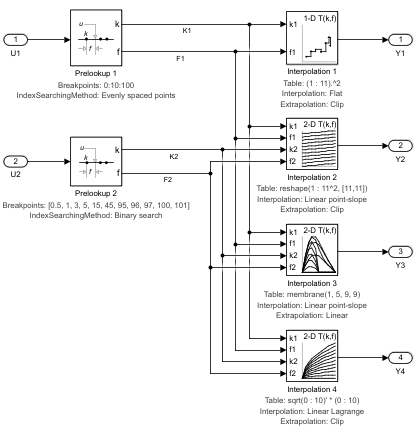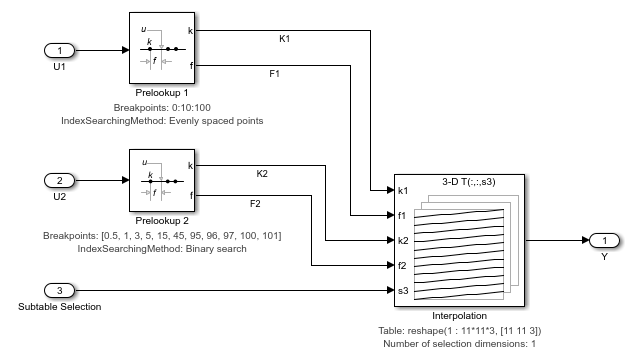Using the Prelookup and Interpolation Blocks

This examples shows how to use the Prelookup and Interpolation Using Prelookup blocks.

Introduction

This model uses Prelookup and Interpolation Using Prelookup blocks. The Prelookup block minimizes the number of index searches performed across a set of lookup tables. It also mixes clipping, extrapolation, and index search algorithms within one table calculation. Note how the Prelookup blocks perform the index search portion of the computation and the Interpolation Using Prelookup blocks perform the rest:These blocks are in the Simulink® block library in the Lookup Tables sublibrary:Optimization: PreLookup and Interpolation Using Prelookup Blocks

Consider using PreLookup block for tables with intensive index searches in breakpoint sets. The Prelookup blocks allow you to perform an index search once and reuse the result in many table lookups. In this model, three index search results are used in five blocks in a total of ten uses. Equivalent regular lookup tables such as the example to the right would have required ten index searches instead of three.Optimization: Subtable Selection Option in the Interpolation Using Prelookup Blocks

One of the interpolation blocks in this model is configured using a capability: trailing dimensions of an n-D table can be marked as "selection dimensions", meaning that the input for that dimension is an integer used only to make a subtable selection, such as picking a 2-D plane from a 3-D table. The subtable is then interpolated normally.

Interpolation of subtables can save tremendous amounts of computation. For every dimension eliminated from interpolation, the computation almost halves. Since an N-dimensional interpolation takes (2^N)-1 individual interpolation operations (y = ylow + f*(yhigh-ylow)), just one dimension of selection can almost double the speed of the interpolation. Extreme example: a 5-D table with three dimensions of subtable selection and 2-D interpolation: 5-D interpolation would take 2^5-1 = 31 interpolations, but a 2-D interpolation takes only 2^2 - 1 = 3.

The selection ports support vectorization to allow multiple subtable selection/interpolations in a single block.The subtable or multitable mode of operation is activated by setting a positive integer for the 'Number of sub-table selections dimensions' parameter in the block parameter dialog. The number you set is interpreted as the number of dimensions to select from the highest dimensions. For example, if you have a 3-D table and choose 2, that means the first dimension will be interpolated and dimensions 2 and 3 will be selected:

NOTE: You can remove highlighting from a model using the View/Remove highlighting menu item or this command:

set_param(mdl,'HiliteAncestors','none')

Breakpoint and Table Data Consistency Checking

Using Prelookup and Interpolation Using Prelookup blocks introduces a need to ensure that the length of the 'Breakpoint data' parameter in the Prelookup blocks matches the corresponding dimension of the 'Table data' parameter in the connected Interpolation Using Prelookup blocks. Simulink® always checks the consistency between the two blocks when updating the block diagram. Simulink reports errors if it encounters inconsistent breakpoint and table data. For example:

try
evalc(['sim(', mdl, ')']);
catch E
disp(['Error:', E.message]);
end
Error:The range of values for input port k2 of block '<a href="matlab:open_and_hilite_hyperlink ('sldemo_bpcheck/Interpolation on an 11x11 table ERROR IN DIM 2 ','error')">sldemo_bpcheck/Interpolation on an 11x11 table ERROR IN DIM 2 </a>' does not match the size of the corresponding table dimension of 11. Specify a different table dimension or modify the range of values for input port k2.